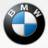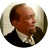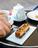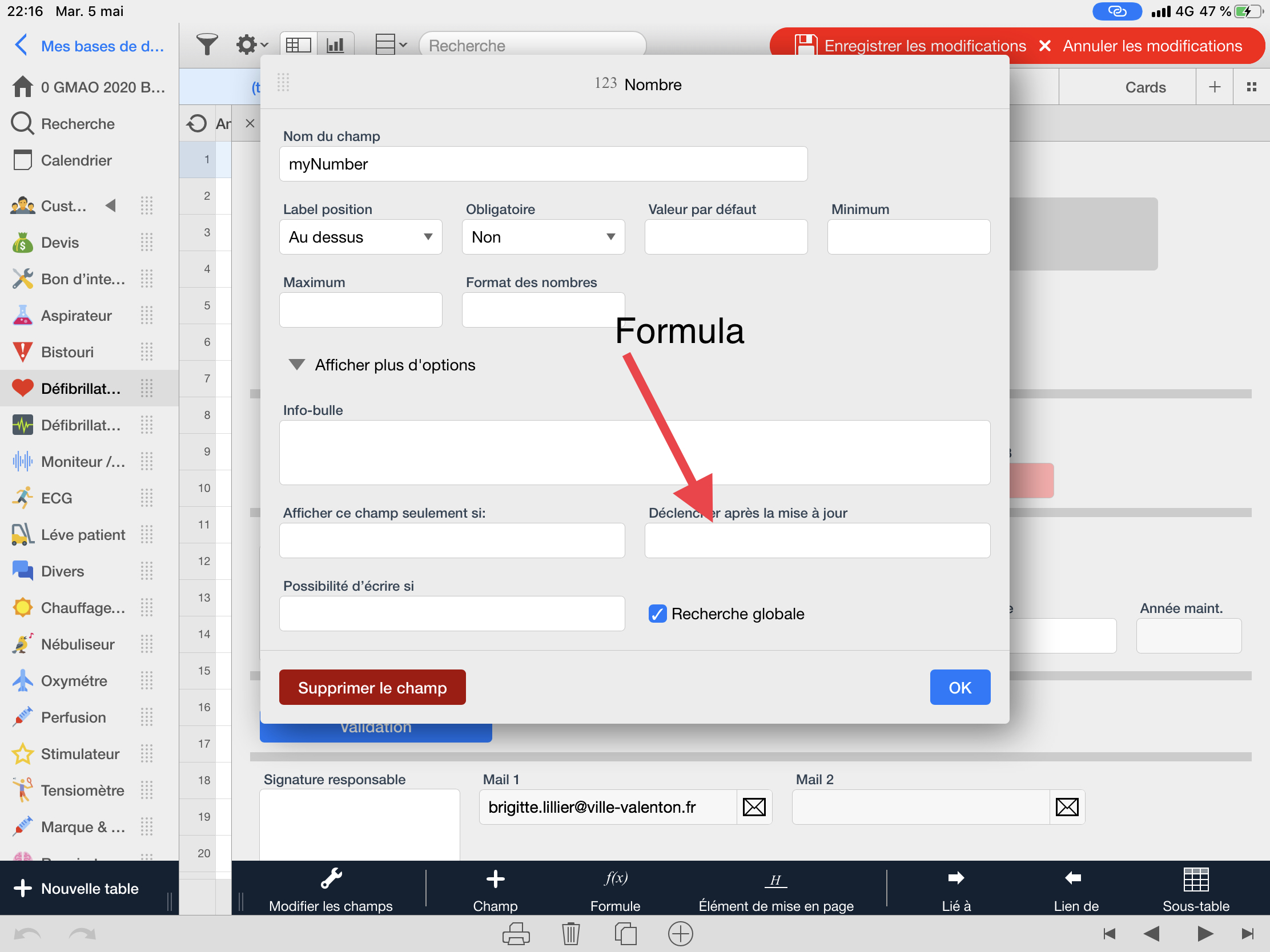Tableview row numberHow can i add row number to my tableview so when i print it out it shows row number, not id numberCreate a new Number field (myNumber).
Use "Mass data update" to populate this field. Use the calculation:
`–––`
`let myID := number(this._id);`
`cnt(select 'Table Name' where number(_id)<=myID)`
`–––`

BirgerHello, not being an expert in programming, I can’t use the formula.
I create the Field myNumber, my table is named 'défibrillateur'
I must replace'Table Name'  with 'défibrillateur' ? and where i put 'myNumber' ?

what would be the exact formula?

i have to put the formula here, I supose ..thanks

alain